# sklearn.preprocessing.PolynomialFeatures¶

class sklearn.preprocessing.PolynomialFeatures(degree=2, *, interaction_only=False, include_bias=True, order='C')[source]

Generate polynomial and interaction features.

Generate a new feature matrix consisting of all polynomial combinations of the features with degree less than or equal to the specified degree. For example, if an input sample is two dimensional and of the form [a, b], the degree-2 polynomial features are [1, a, b, a^2, ab, b^2].

Read more in the User Guide.

Parameters:
degreeint or tuple (min_degree, max_degree), default=2

If a single int is given, it specifies the maximal degree of the polynomial features. If a tuple (min_degree, max_degree) is passed, then min_degree is the minimum and max_degree is the maximum polynomial degree of the generated features. Note that min_degree=0 and min_degree=1 are equivalent as outputting the degree zero term is determined by include_bias.

interaction_onlybool, default=False

If True, only interaction features are produced: features that are products of at most degree distinct input features, i.e. terms with power of 2 or higher of the same input feature are excluded:

• included: x, x, x * x, etc.

• excluded: x ** 2, x ** 2 * x, etc.

include_biasbool, default=True

If True (default), then include a bias column, the feature in which all polynomial powers are zero (i.e. a column of ones - acts as an intercept term in a linear model).

order{‘C’, ‘F’}, default=’C’

Order of output array in the dense case. 'F' order is faster to compute, but may slow down subsequent estimators.

New in version 0.21.

Attributes:
powers_ndarray of shape (n_output_features_, n_features_in_)

Exponent for each of the inputs in the output.

n_features_in_int

Number of features seen during fit.

New in version 0.24.

feature_names_in_ndarray of shape (n_features_in_,)

Names of features seen during fit. Defined only when X has feature names that are all strings.

New in version 1.0.

n_output_features_int

The total number of polynomial output features. The number of output features is computed by iterating over all suitably sized combinations of input features.

SplineTransformer

Transformer that generates univariate B-spline bases for features.

Notes

Be aware that the number of features in the output array scales polynomially in the number of features of the input array, and exponentially in the degree. High degrees can cause overfitting.

Examples

>>> import numpy as np
>>> from sklearn.preprocessing import PolynomialFeatures
>>> X = np.arange(6).reshape(3, 2)
>>> X
array([[0, 1],
[2, 3],
[4, 5]])
>>> poly = PolynomialFeatures(2)
>>> poly.fit_transform(X)
array([[ 1.,  0.,  1.,  0.,  0.,  1.],
[ 1.,  2.,  3.,  4.,  6.,  9.],
[ 1.,  4.,  5., 16., 20., 25.]])
>>> poly = PolynomialFeatures(interaction_only=True)
>>> poly.fit_transform(X)
array([[ 1.,  0.,  1.,  0.],
[ 1.,  2.,  3.,  6.],
[ 1.,  4.,  5., 20.]])


Methods

 fit(X[, y]) Compute number of output features. fit_transform(X[, y]) Fit to data, then transform it. get_feature_names_out([input_features]) Get output feature names for transformation. Get metadata routing of this object. get_params([deep]) Get parameters for this estimator. set_output(*[, transform]) Set output container. set_params(**params) Set the parameters of this estimator. Transform data to polynomial features.
fit(X, y=None)[source]

Compute number of output features.

Parameters:
X{array-like, sparse matrix} of shape (n_samples, n_features)

The data.

yIgnored

Not used, present here for API consistency by convention.

Returns:
selfobject

Fitted transformer.

fit_transform(X, y=None, **fit_params)[source]

Fit to data, then transform it.

Fits transformer to X and y with optional parameters fit_params and returns a transformed version of X.

Parameters:
Xarray-like of shape (n_samples, n_features)

Input samples.

yarray-like of shape (n_samples,) or (n_samples, n_outputs), default=None

Target values (None for unsupervised transformations).

**fit_paramsdict

Returns:
X_newndarray array of shape (n_samples, n_features_new)

Transformed array.

get_feature_names_out(input_features=None)[source]

Get output feature names for transformation.

Parameters:
input_featuresarray-like of str or None, default=None

Input features.

• If input_features is None, then feature_names_in_ is used as feature names in. If feature_names_in_ is not defined, then the following input feature names are generated: ["x0", "x1", ..., "x(n_features_in_ - 1)"].

• If input_features is an array-like, then input_features must match feature_names_in_ if feature_names_in_ is defined.

Returns:
feature_names_outndarray of str objects

Transformed feature names.

Get metadata routing of this object.

Please check User Guide on how the routing mechanism works.

Returns:

A MetadataRequest encapsulating routing information.

get_params(deep=True)[source]

Get parameters for this estimator.

Parameters:
deepbool, default=True

If True, will return the parameters for this estimator and contained subobjects that are estimators.

Returns:
paramsdict

Parameter names mapped to their values.

property powers_

Exponent for each of the inputs in the output.

set_output(*, transform=None)[source]

Set output container.

See Introducing the set_output API for an example on how to use the API.

Parameters:
transform{“default”, “pandas”}, default=None

Configure output of transform and fit_transform.

• "default": Default output format of a transformer

• "pandas": DataFrame output

• None: Transform configuration is unchanged

Returns:
selfestimator instance

Estimator instance.

set_params(**params)[source]

Set the parameters of this estimator.

The method works on simple estimators as well as on nested objects (such as Pipeline). The latter have parameters of the form <component>__<parameter> so that it’s possible to update each component of a nested object.

Parameters:
**paramsdict

Estimator parameters.

Returns:
selfestimator instance

Estimator instance.

transform(X)[source]

Transform data to polynomial features.

Parameters:
X{array-like, sparse matrix} of shape (n_samples, n_features)

The data to transform, row by row.

Prefer CSR over CSC for sparse input (for speed), but CSC is required if the degree is 4 or higher. If the degree is less than 4 and the input format is CSC, it will be converted to CSR, have its polynomial features generated, then converted back to CSC.

If the degree is 2 or 3, the method described in “Leveraging Sparsity to Speed Up Polynomial Feature Expansions of CSR Matrices Using K-Simplex Numbers” by Andrew Nystrom and John Hughes is used, which is much faster than the method used on CSC input. For this reason, a CSC input will be converted to CSR, and the output will be converted back to CSC prior to being returned, hence the preference of CSR.

Returns:
XP{ndarray, sparse matrix} of shape (n_samples, NP)

The matrix of features, where NP is the number of polynomial features generated from the combination of inputs. If a sparse matrix is provided, it will be converted into a sparse csr_matrix.

## Examples using sklearn.preprocessing.PolynomialFeatures¶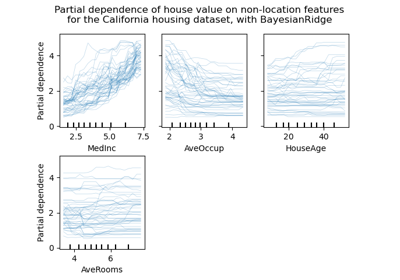Release Highlights for scikit-learn 0.24

Release Highlights for scikit-learn 0.24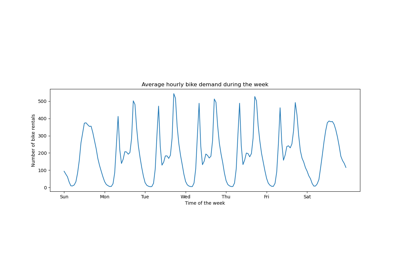Time-related feature engineering

Time-related feature engineering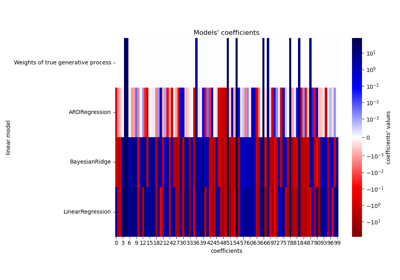Comparing Linear Bayesian Regressors

Comparing Linear Bayesian Regressors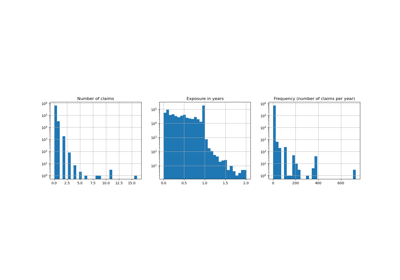Poisson regression and non-normal loss

Poisson regression and non-normal loss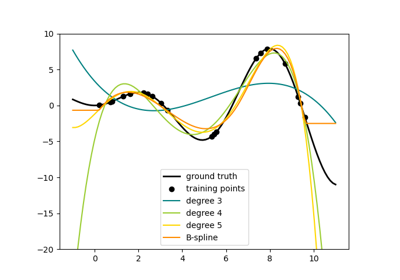Polynomial and Spline interpolation

Polynomial and Spline interpolation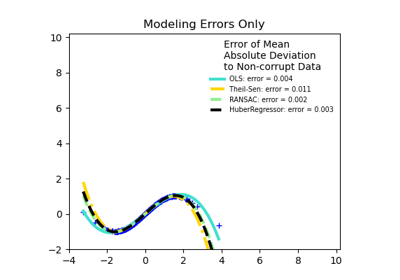Robust linear estimator fitting

Robust linear estimator fittingDisplaying Pipelines

Displaying Pipelines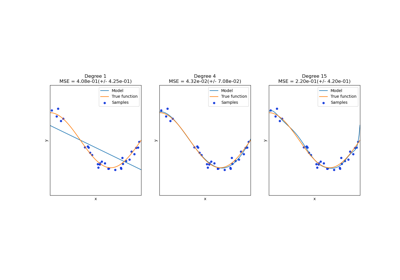Underfitting vs. Overfitting

Underfitting vs. Overfitting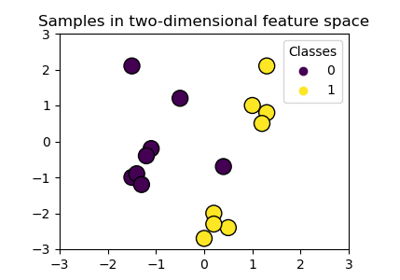Plot classification boundaries with different SVM Kernels

Plot classification boundaries with different SVM Kernels